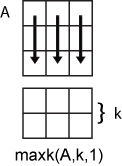# maxk

Find `k` largest elements of array

## Syntax

``B = maxk(A,k)``
``B = maxk(A,k,dim)``
``B = maxk(___,'ComparisonMethod',c)``
``[B,I] = maxk(___)``

## Description

example

````B = maxk(A,k)` returns the `k` largest elements of `A`. If `A` is a vector, then `maxk` returns a vector containing the `k` largest elements of `A`.If `A` is a matrix, then `maxk` returns a matrix whose columns contain the `k` largest elements of each column of `A`.If `A` is a multidimensional array, then `maxk` returns the `k` largest elements along the first dimension whose size does not equal 1. ```

example

````B = maxk(A,k,dim)` determines the `k` largest elements of `A` along dimension `dim`.```

example

````B = maxk(___,'ComparisonMethod',c)` optionally specifies how to compare elements of `A` for any of the previous syntaxes. For example, `maxk(A,k,'ComparisonMethod','abs')` returns the `k` largest elements of `A` according to their absolute values.```

example

````[B,I] = maxk(___)` finds the indices of the largest `k` values of `A` and returns them in `I`.```

## Examples

collapse all

Compute the largest 3 elements of a vector.

```A = 1:10; B = maxk(A,3)```
```B = 1×3 10 9 8 ```

Compute the largest 3 elements of each row of a matrix.

`A = magic(5)`
```A = 5×5 17 24 1 8 15 23 5 7 14 16 4 6 13 20 22 10 12 19 21 3 11 18 25 2 9 ```
`B = maxk(A,3,2)`
```B = 5×3 24 17 15 23 16 14 22 20 13 21 19 12 25 18 11 ```

Compute the 2 largest elements of a complex vector according to their magnitude, and return the indices where they are located in the input vector.

`A = [2-2i 5+i -7-3i -1+i]`
```A = 1×4 complex 2.0000 - 2.0000i 5.0000 + 1.0000i -7.0000 - 3.0000i -1.0000 + 1.0000i ```
`[B,I] = maxk(A,2,'ComparisonMethod','abs')`
```B = 1×2 complex -7.0000 - 3.0000i 5.0000 + 1.0000i ```
```I = 1×2 3 2 ```

## Input Arguments

collapse all

Input array, specified as a vector, matrix, or multidimensional array.

• If `A` is a vector, then `maxk` returns a vector containing the `k` largest elements of `A`.

• If `A` is a matrix, then `maxk` returns a matrix whose columns contain the `k` largest elements of each column of `A`.

• If `A` is a multidimensional array, then `maxk` returns the `k` largest elements along the first dimension whose size does not equal 1.

If `A` has type `categorical`, then it must be ordinal.

Data Types: `single` | `double` | `int8` | `int16` | `int32` | `int64` | `uint8` | `uint16` | `uint32` | `uint64` | `logical` | `char` | `categorical` | `datetime` | `duration`
Complex Number Support: Yes

Number of maxima to return, specified as a positive integer scalar. If `k` is greater than or equal to the number of elements in the operating dimension, then `maxk` sorts the input array along that dimension.

Operating dimension, specified as a positive integer scalar. If no value is specified, then the default is the first array dimension whose size does not equal 1.

Consider an `m`-by-`n` input matrix, `A`:

• `maxk(A,k,1)` computes the `k` largest values in each column of `A` and returns a `k`-by-`n` matrix.• `maxk(A,k,2)` computes the `k` largest values in each row of `A` and returns an `m`-by-`k` matrix.Comparison method, specified as one of the following:

• `'auto'` — Compare elements of input `A` by `real(A)` when `A` is real, and by `abs(A)` when `A` is complex.

• `'real'` — Compare elements of input `A` by `real(A)` when `A` is real or complex. If `A` has elements with equal real parts, then use `imag(A)` to break ties.

• `'abs'` — Compare elements of input `A` by `abs(A)` when `A` is real or complex. If `A` has elements with equal magnitude, then use `angle(A)` in the interval (-π,π] to break ties.

## Output Arguments

collapse all

Output array, returned as a scalar, vector, matrix, or multidimensional array. `maxk` returns the `k` elements in order from largest to smallest. The order of the elements in `B` preserves the order of any equal elements in `A`.

Index array, returned as a vector, matrix, or multidimensional array. `I` is the same size as `B`. If the output array `B` contains repeated elements, then the order of their indices in `I` matches the order in which they appear in the input array.

## Version History

Introduced in R2017b